Two trains

There were 159 freight wagons on the railway station creating 2 trains. One had 15 more wagons than the other. How many wagons did each train have?

Result

a =  87
b =  72

Solution:

a+b = 159
a = 15+b

a+b = 159
a-b = 15

a = 87
b = 72

Calculated by our linear equations calculator.

Leave us a comment of example and its solution (i.e. if it is still somewhat unclear...):Be the first to comment!To solve this example are needed these knowledge from mathematics:

Do you have a linear equation or system of equations and looking for its solution? Or do you have quadratic equation?

Next similar examples:

1. Boys and girlsThere are 48 children in the sports club, boys are 10 more than girls. How many girls go to the club?
2. Boys and girls 2The ratio of boys to girls in math club is 4:3 . After 8 more girls joined the Club, the ratio become 1:1. How many members are there in the club now?
3. Bottles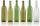Mrs. Violet brewed 15 l of raspberry juice. She poured it into 17 bottles. Some were 3/4 liter and some one liter. How many was bottles of this two types?
4. Translations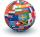If I going to translate the book 6 pages per day I translate it 4 days earlier than if I translated 5 pages a day. If I translate 4 pages a day I translate it for how many days.....?
5. Spain vs USASpain lost to the US by 4 goals. In the match total fell 10 goals. How many goals gave the Spain and how the United States?
6. Linear systemSolve this linear system (two linear equations with two unknowns): x+y =36 19x+22y=720
7. Football match 4In a football match with the Italy lost 3 goals with Germans. Totally fell 5 goals in the match. Determine the number of goals of Italy and Germany.
8. EquationSolve the equation: 1/2-2/8 = 1/10; Write the result as a decimal number.
9. Unknown numberIdentify unknown number which 1/5 is 40 greater than one tenth of that number.
10. Simple equationSolve for x: 3(x + 2) = x - 18
11. Tickets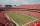On the football tournament ticket cost 45 Kc for standing and 120kč for sitting. Sitting spectators was 1/3 more than standing. The organizers collected a total 12 300 Kc. How many seated and standing seats (spectators)?
12. Tippler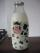Bottle with cork cost 8.8 Eur. The bottle is 0.8 euros more expensive than cork. How much is a bottle and the cork?
13. Parking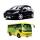120 vehicles parking on the morning. Pasenger car is charged 20 CZK, 50 CZK per bus. The guard collected for parking 2640 CZK in total. How many cars and how many buses stood in the parking?
14. Every day 7 pagesAdelka reads the book every day 7 pages. When she reads one more page a day she will read it three days earlier. How long will Adelka read a book? How much does a book of pages have?
15. Two numbers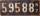Determine the numbers x and y so x + y = 8 is truth and the numbers are in the ratio of 4: 5.Number 118 divide into two addends, so first addend is 69 greater than 75% of the second addend.Half a kilogram of pork and three-quarters of a kilogram of beef cost total 5.1 USD. One quarter of a kilogram of beef and 1 kilogram of pork cost 4.9 USD. Determine the price of 1 kg of each type of meat.